# Zonal Statistics and Trial Plots Analytics

### Introduction

Zonal Statistics tool makes it easy and efficient to analyse specific areas of the field and get a number of metrics per vegetation index and per band. Zonal Statistics work in any type of field and imagery, but it's especially useful in fields with trial areas as it provides tools to identify boundaries of each individual plot and calculate statistics for it.

### Trial plots

In order to identify boundaries of each individual plot in the trial area, boundaries of the whole trial area and of 4 neighbouring plots in any corner of the plot area needs to be provided. Assuming that the shape and distance between the plots and rows doesn't vary significantly, all other plots in the area will be identified and a polygon will be placed within the safe distance from the edges of each plot. When calculating statistics in the next step, only the the area within the polygons will be used. Steps below describe the process of defining the trial area and detecting plot boundaries.

1. Open imagery of interest, switch to Analysys & Prescription tab and activate Zonal Statistics tool: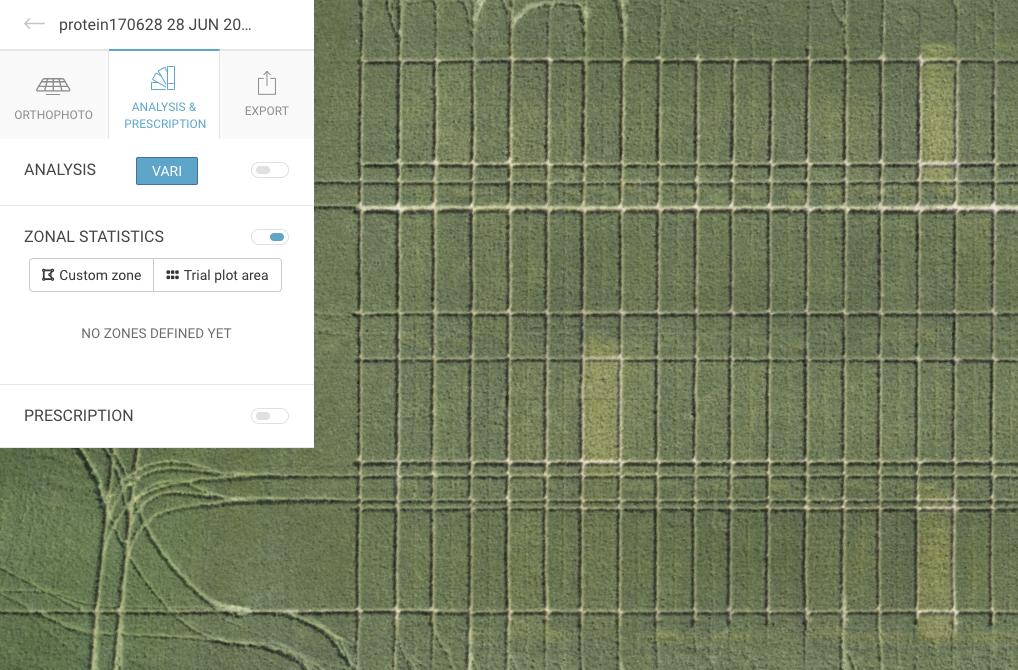2. Click on the button "Trial plot area" and a template for the required input will appear on the map: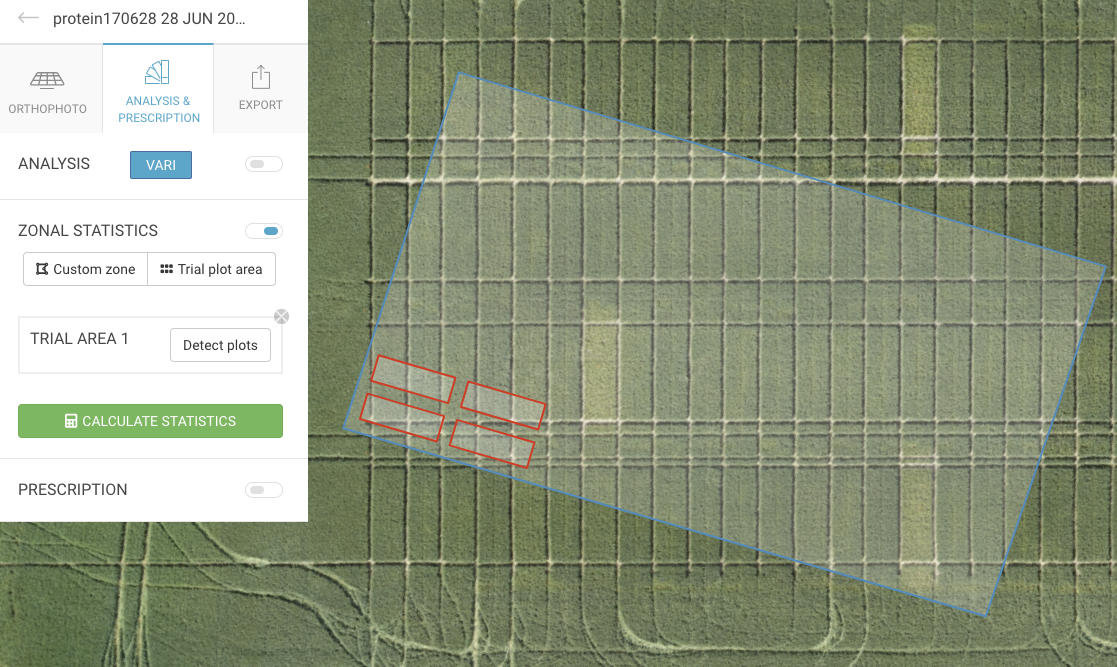3. Click once on the blue polygon to make it editable, then drag its edges to the corners of the whole trial plot area. Try to place those edges as accurately as possible on the corners of edging plots: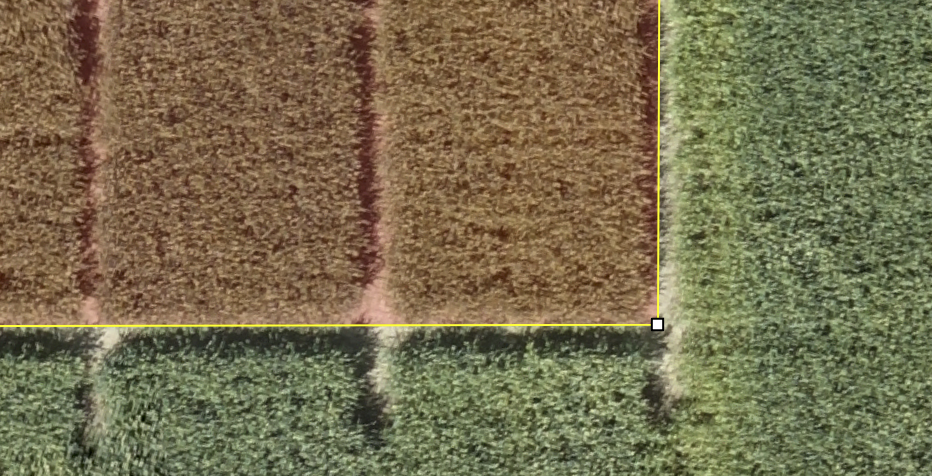4. Now do the same with the red polygons to outline boundaries for 4 neighbouring plots in any corner of the trial area. Notice that each plot will be assigned an unique id starting from the corner where you provide plot examples so place those examples where you want the numbering to start. Final input should look like this: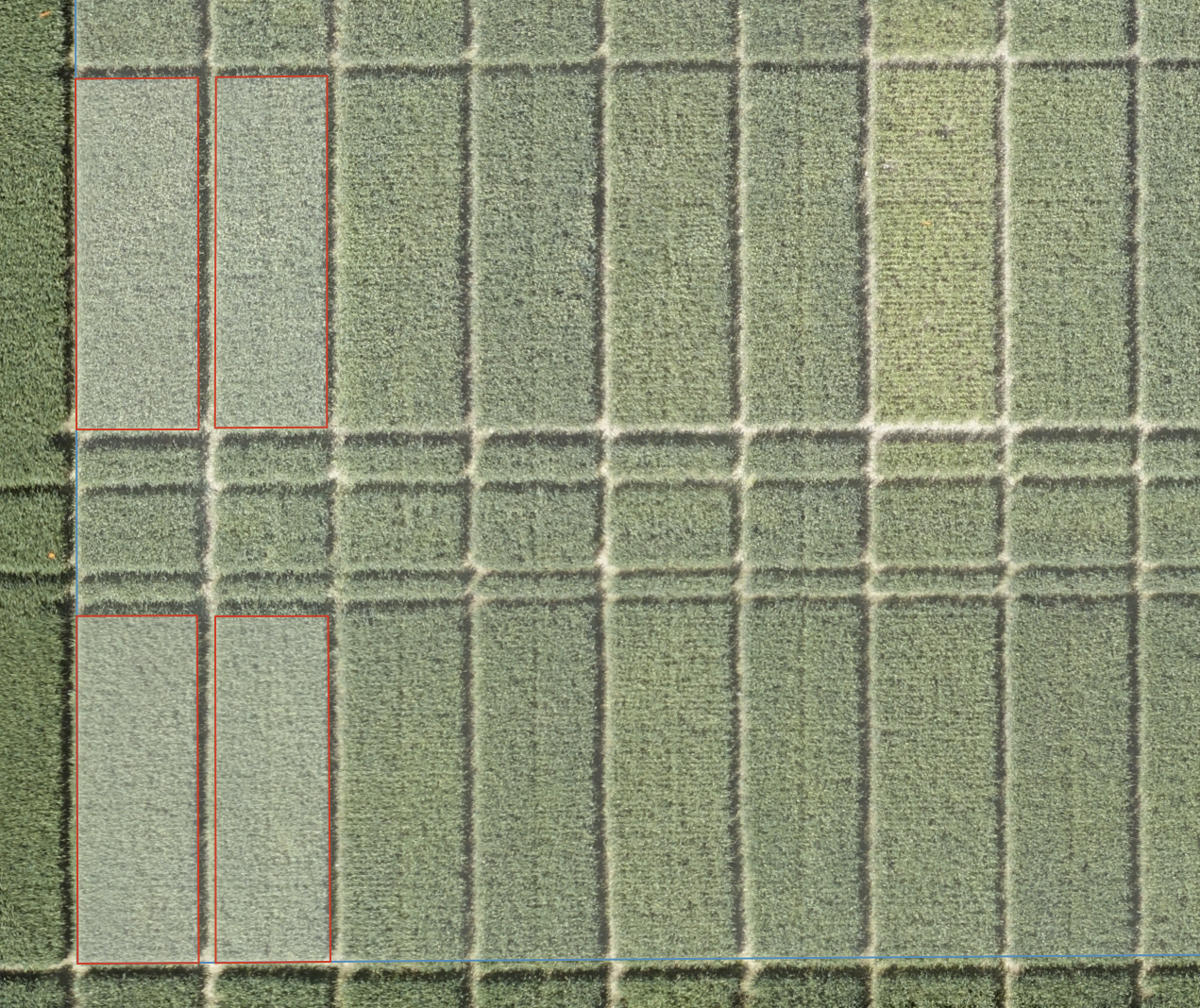5. Now click on "Detect plots" button in the left panel to detect remaining plots. This process might take a while depending on the size of the trial area and imagery resolution. When ready, detected plots will be displayed as black polygons with a label showing assigned id. If certain polygon is not placed correctly, its position and size can easily be adjusted - just select polygon of interest to make it editable and drag it and/or adjust its edges as needed. If there are several trial areas in the field, the process can be repeated from Step 2.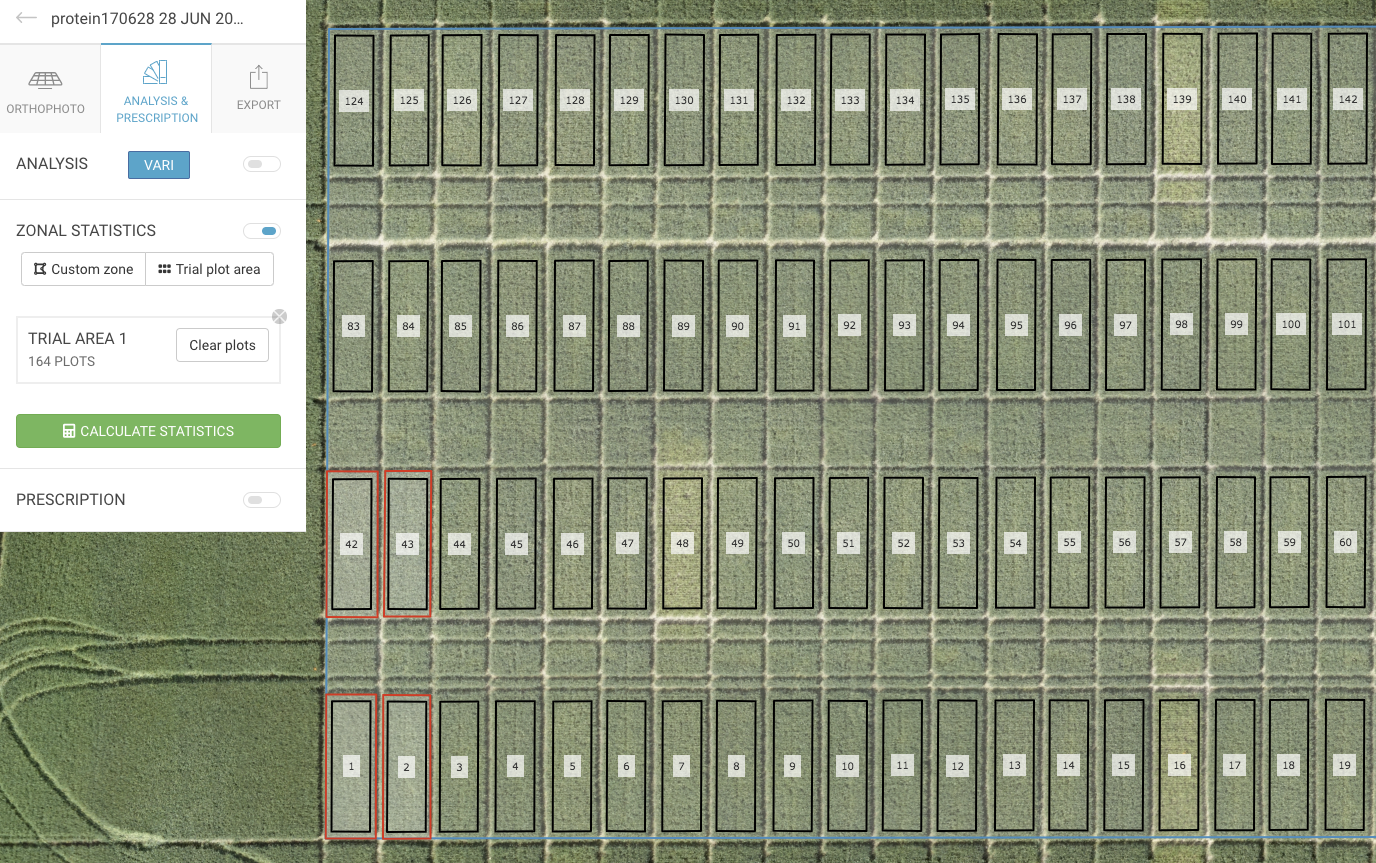### Custom Zones

In addition to trial areas, one or more custom zones of any shape can be defined in the fields. To do that, click on the "Custom zone" button in Zonal Statistics tool and draw a polygon by clicking on a map to define zone boundaries. Click on the first point of the polygon or double click to finish the drawing. Repeat the process to add new custom zones. Every zone can be edited the same way as trial plots - just click on it once to make it editable.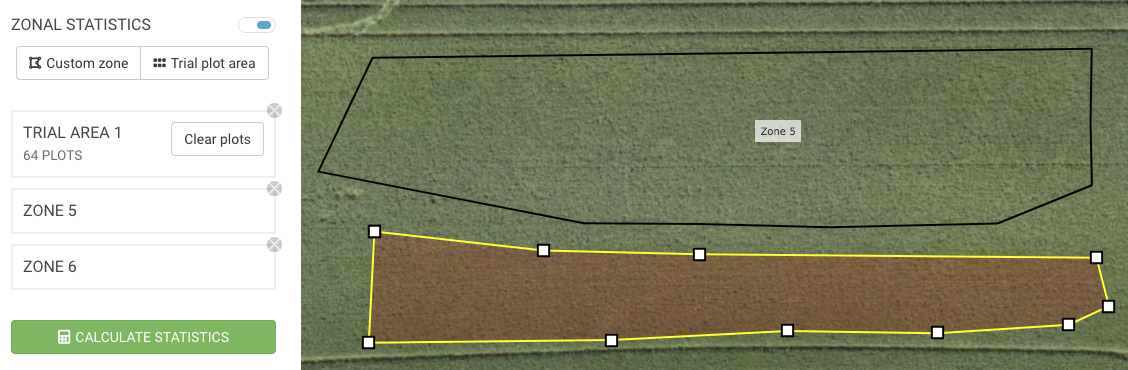### Calculating statistics

In order to calculate statistics click on the green "Calculate statistics" button at the bottom of Zonal Statistic tool. This process may take a while depending on number of zones/plots. When done, a mean value will be displayed below the id label of each plot/zone. Apart from the mean value a number of other metrics is calculated for the selected vegetation index as well as raw band values. These metrics can be accessed by exporting a CSV-file which is described in the next step.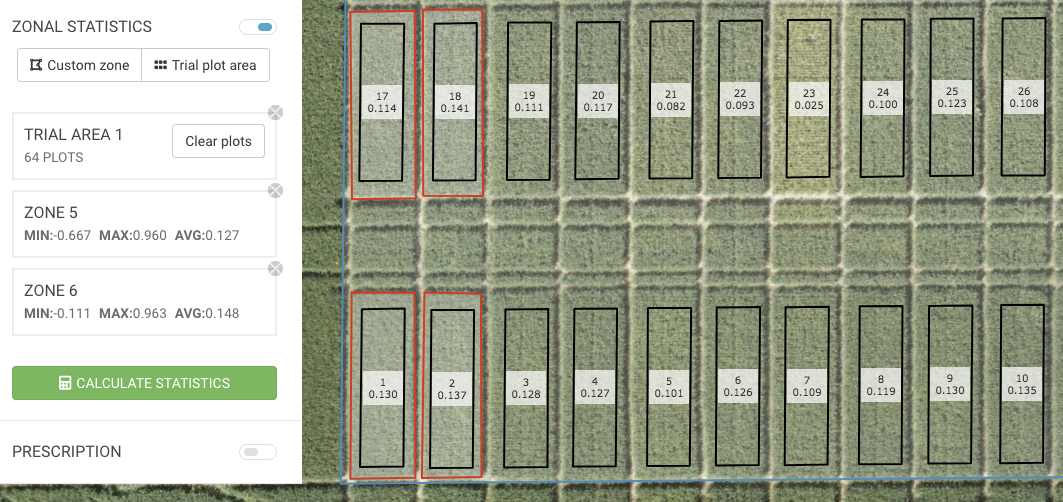Apart from the median vegetation index value that is displayed on the map, a number of other metrics are calculated for every plot or custom zone:

• Minimum
• Maximum
• Mean
• Median
• Standard deviation
• 25th and 75th percentile
• Number of pixels used in calculation

Metrics above are calculated for the vegetation index selected in "Analysis"-section, as well as for raw pixel values of each individual band.

Exporting statistics

Zonal Statistics can be exported in 2 different formats:

• ESRI Shape file containing boundaries of each plot/zone in WGS84 coordinate system along with calculated metrics as attributes. Can be useful if there a need for further analysis in software like QGIS or ArcGIS
• CSV file containing plot/zone id, statistics for selected vegetation index as well as for each individual band found in the imagery

To export statistics switch to the Export-tab, toggle Zonal Statistics- dropdown, select format and click on Zonal Statistics-button to download.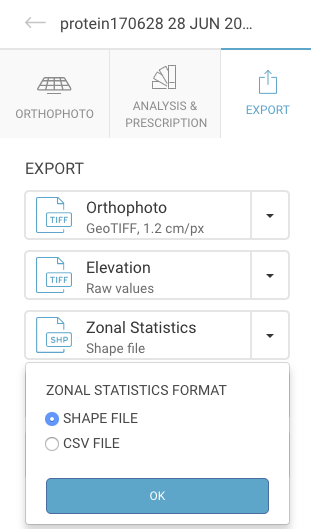The trial plot extraction tool has been developed within the SLU programme Laboratory for Intelligent Decision Support Systems (LADS) and with financial support from Vinnova(dnr: 2016–04248).